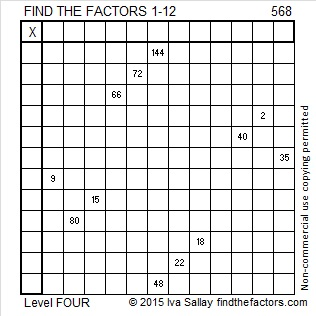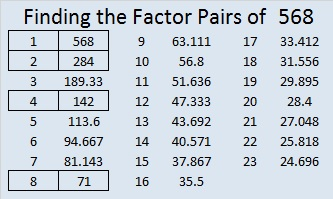# 568 and Level 4

568 is the sum of the first 19 prime numbers (all the primes from 2 to 67).Print the puzzles or type the solution on this excel file: 12 Factors 2015-07-27

—————————————————————————————————

• 568 is a composite number.
• Prime factorization: 568 = 2 x 2 x 2 x 71, which can be written 568 = (2^3) x 71
• The exponents in the prime factorization are 3 and 1. Adding one to each and multiplying we get (3 + 1)(1 + 1) = 4 x 2 = 8. Therefore 568 has exactly 8 factors.
• Factors of 568: 1, 2, 4, 8, 71, 142, 284, 568
• Factor pairs: 568 = 1 x 568, 2 x 284, 4 x 142, or 8 x 71
• Taking the factor pair with the largest square number factor, we get √568 = (√4)(√142) = 2√142 ≈ 23.83275—————————————————————————————————This site uses Akismet to reduce spam. Learn how your comment data is processed.